# GPS Waveform Generation

This example shows how to generate Global Positioning System (GPS) legacy navigation (LNAV) data, civil navigation (CNAV) data, and a complex baseband waveform. The spreading of the data is performed with coarse acquisition code (C/A-code), precision code (P-code), or civil moderate / civil long code (L2 CM-/L2 CL-code). This example shows GPS waveform generation according to the IS-GPS-200L standard . To design a navigation system based on GPS, you must test the receiver with a received signal. Because you cannot control transmitter and channel parameters, a signal that is received from a satellite is not useful for testing a receiver. To test the receiver, you must use a waveform that is generated under a controlled set of parameters.

### Introduction

Generate a GPS signal by using these three steps.

1. Generate GPS data bits by using the configuration parameters that are described in later sections. The data bits are generated at a rate of 50 bits per second (bps).

2. Spread these low rate data bits by using high rate spreading codes. The GPS standard  specifies three kinds of spreading codes: C/A-code, P-code, and L2 CM-/L2 CL-code. In addition to these three codes, this standard also specifies Y-code to use instead of P-code when anti-spoofing mode of an operation is active. P-code and Y-code together are called P(Y)-code. The configuration parameters determine the spreading code that is used to generate the waveform.

3. Generate the GPS complex baseband waveform from the bits that are spread by the spreading codes by selecting codes on the in-phase branch and quadrature-phase branch according to the set of configuration parameters.

This figure shows the block diagram of the GPS waveform generator from the configuration parameters.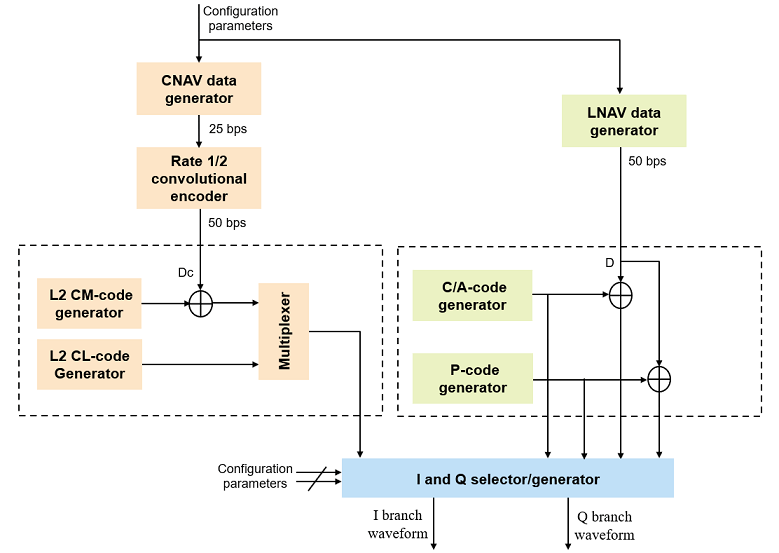### GPS Signal Structure

The standard  describes the transmission of GPS signals on two frequencies: L1 (1575.42 MHz) and L2 (1227.60 MHz). A signal of base frequency 10.23 MHz generates both of these signals. The signal frequency of L1 is 154 x 10.23 MHz = 1575.42 MHz, and the signal frequency of L2 is 120 x 10.23 MHz = 1227.60 MHz. The code-division-multiple-access technique enables you to differentiate between the satellites even though all GPS satellites transmit on the same frequency.

With the evolution of GPS, the signal structure is expanded to improve the navigation performance. For instance, LNAV data is transmitted on the L1-band from the inception of GPS. From GPS block IIR-M onwards, CNAV data is transmitted on the L2-band in addition to the LNAV data that exists on both L1 and L2 bands. This table shows these signal configurations and GPS evolution. "Dc" represents the CNAV data bits and "D" represents the LNAV data bits. Bit operator, $\oplus$, represents the XOR operation.Using this table to choose the content that needs to be transmitted in the in-phase and quadrature-phase branches.

`IBranchContent =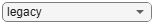"P(Y) + D"`
```IBranchContent = "P(Y) + D" ```
`QBranchContent =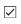"C/A + D"`
```QBranchContent = "C/A + D" ```

Many of the transmitted properties in the LNAV and CNAV data are same, but the frame structure is different. The LNAV data is transmitted in 1500 bit-length frames, with each frame consisting of five subframes of 300 bits in each subframe. Because the data rate is 50 bps, transmitting each subframe takes 6 seconds and transmitting each frame takes 30 seconds. Each subframe consists of 10 words with 30 bits (24 data bits and 6 parity bits) in each word. The GPS data contains information regarding the clock and the position of the satellites. This figure shows the frame structure of the LNAV data.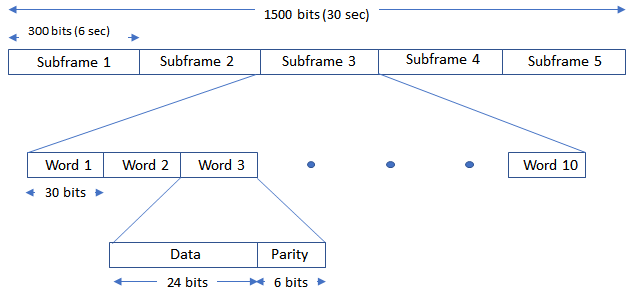The CNAV data is transmitted continuously in the form of message types. Each message type consists of 300 bits that are transmitted at 25 bps. These bits are passed through a rate-half convolutional-encoder to obtain 600 bits from each message type at 50 bps. Transmitting each message type takes 12 seconds. The standard  defines 14 message types in this order: 10, 11, 30, 31, 32, 33, 34, 35, 36, 37, 12, 13, 14, and 15. For a detailed description of each message type and the data transmitted, see IS-GPS-200L Appendix III . The order in which each message type is transmitted is completely arbitrary but is sequenced to provide optimal user experience. In this example, you can choose the order in which these message types are transmitted. This figure shows the CNAV message structure.The message type shown in this figure has these fields:

• PRN ID: Pseudo-random noise (PRN) index

• MSG: Message

• TOW: Time of week

• CRC: Cyclic redundancy check

Set the `ShowVisualizations` property to enable the spectrum and correlation plot visualizations. Set the `WriteWaveformToFile` property to write the complex baseband waveform to a file if needed. This example enables visualizations and disables writing the waveform to a file.

```ShowVisualizations =true; WriteWaveformToFile =false;```

Specify the satellite PRN index as an integer in the range [1,63].

`PRNID = 1;`

Because generating the GPS waveform for the entire navigation data can take a lot of time and memory, this example demonstrates generating a waveform for only one bit of the navigation data. You can control generating the waveform for a specified number of data bits by using the property `NumNavDataBits`.

```% Set this value to 1 to generate the waveform from the first bit of the % navigation data NavDataBitStartIndex = 1321; % Set this value to control the number of navigation data bits in the % generated waveform NumNavDataBits = 1;```

### GPS Data Initialization

Initialize the data configuration object to generate the CNAV data. You can create a configuration object from the `HelperGPSNavigationConfig` object. Update the configuration object properties to customize the waveform as required.

`cnavConfig = HelperGPSNavigationConfig("DataType","CNAV","PRNID",PRNID)`
```cnavConfig = HelperGPSNavigationConfig with properties: DataType: "CNAV" PRNID: 1 MessageTypes: [4x15 double] Preamble: 139 HOWTOW: 1 L2CPhasing: 0 CEIDataSet: [1x1 HelperGPSCEIConfig] AgeOfDataOffset: 0 AlmanacFileName: "gpsAlmanac.txt" Ionosphere: [1x1 struct] EarthOrientation: [1x1 struct] UTC: [1x1 struct] DifferentialCorrection: [1x1 struct] TimeOffset: [1x1 struct] TextInMessageType36: 'This content is part of Satellite Communications Toolbox' TextInMessageType15: 'This content is part of Satellite Communications Toolbox' ```

The property, `CEIDataSet`, is a configuration object of type `HelperGPSCEIConfig`, which contains clock, ephemeris, and integrity (CEI) data parameters.

In theory, clocks of all satellites must be synchronized, which implies that all GPS satellite clocks must show the same time at a given moment in time. In practice, deterministic satellite clock error characteristics of bias, drift, and aging as well as satellite implementation characteristics of group delay bias and mean differential group delay exist. These errors deviate the satellite clocks from the GPS system time.

GPS satellites revolve around the Earth in an elliptical orbit, with Earth at one of the focal points of the ellipse. A set of orbital parameters that accurately defines a satellite position in this elliptical orbit is called ephemeris. Each GPS satellite transmits its own ephemeris data on subframes 2 and 3 for LNAV data and on message types 10 and 11 for CNAV data. This figure shows five orbital parameters for a satellite in the Earth-centered Earth-fixed (ECEF) coordinate system. This figure does not show an actual GPS satellite, and the figure is for illustration only.

• Length of semimajor axis, $\mathit{A}$: Distance from the center of the elliptical orbit of the satellite to the apogee or perigee

• Inclination angle, $\mathit{i}$: Angle between the equatorial plane of Earth and the plane of the orbit of the satellite

• Longitude of ascending node, $\Omega$: Angle between the Greenwich meridian and the direction of the ascending node

• Argument of perigee, $\omega$: Angle between the direction of the ascending node and the direction of the perigee

• True anomaly, $\upsilon$: Angle between the direction of the perigee and the direction of the current position of the satellite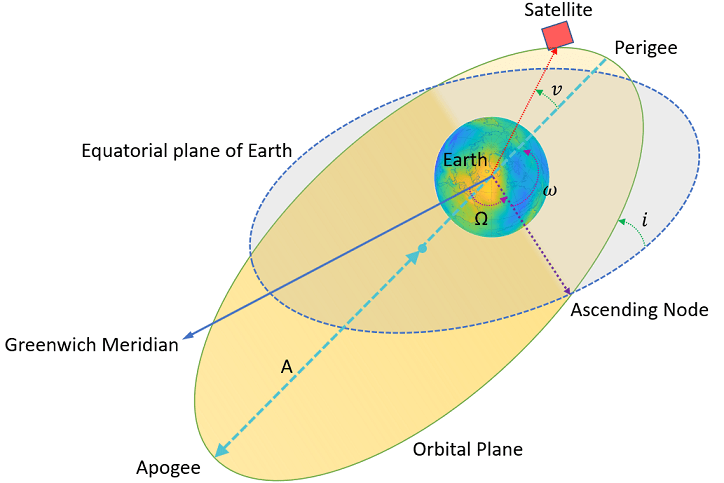Per Kepler's second law of planetary motion, the angular velocity (rate of change of true anomaly) is different at different locations in the orbit. You can define the mean anomaly, whose rate of change is constant over the entire orbit of the satellite. In GPS ephemeris parameters, rather than specifying true anomaly, mean anomaly is specified (from which true anomaly can be found). IS-GPS-200L Table 20-IV  specifies the algorithms that relate mean anomaly and true anomaly.

The eccentricity of the ellipse also defines the orbit. Eccentricity gives a measure of deviation of the elliptical orbit from the circular shape.

This command displays the CEI data set properties that are related to the CNAV data.

`cnavConfig.CEIDataSet`
```ans = HelperGPSCEIConfig with properties: SignalHealth: [3x1 double] WeekNumber: 2149 GroupDelayDifferential: 0 SVClockCorrectionCoefficients: [3x1 double] ReferenceTimeOfClock: 0 SemiMajorAxisLength: 26560 ChangeRateInSemiMajorAxis: 0 MeanMotionDifference: 0 RateOfMeanMotionDifference: 0 Eccentricity: 0.0200 MeanAnomaly: 0 ReferenceTimeOfEphemeris: 0 HarmonicCorrectionTerms: [6x1 double] IntegrityStatusFlag: 0 ArgumentOfPerigee: -0.5200 RateOfRightAscension: 0 LongitudeOfAscendingNode: -0.8400 Inclination: 0.3000 InclinationRate: 0 URAEDID: 0 InterSignalCorrection: [4x1 double] ReferenceTimeCEIPropagation: 0 ReferenceWeekNumberCEIPropagation: 101 URANEDID: [3x1 double] AlertFlag: 0 ```

A similar set of properties exist for the LNAV data. Create a configuration object to store the LNAV data.

`lnavConfig = HelperGPSNavigationConfig("DataType","LNAV","PRNID",PRNID)`
```lnavConfig = HelperGPSNavigationConfig with properties: DataType: "LNAV" PRNID: 1 FrameIndices: [1 2 3 4 5 6 7 8 9 10 11 12 13 14 15 16 ... ] Preamble: 139 TLMMessage: 0 HOWTOW: 1 AntiSpoofFlag: 0 CodesOnL2: "P-code" L2PDataFlag: 0 CEIDataSet: [1x1 HelperGPSCEIConfig] AgeOfDataOffset: 0 NMCTAvailabilityIndicator: 0 NMCTERD: [30x1 double] AlmanacFileName: "gpsAlmanac.txt" Ionosphere: [1x1 struct] UTC: [1x1 struct] TextMessage: 'This content is part of Satellite Communications Toolbox' ```

Similar to the CNAV data, the LNAV data has CEI data properties that are different from that of CNAV. This command displays the LNAV CEI data properties.

`lnavConfig.CEIDataSet`
```ans = HelperGPSCEIConfig with properties: SVHealth: 0 IssueOfDataClock: 0 URAID: 0 WeekNumber: 2149 GroupDelayDifferential: 0 SVClockCorrectionCoefficients: [3x1 double] ReferenceTimeOfClock: 0 SemiMajorAxisLength: 26560 MeanMotionDifference: 0 FitIntervalFlag: 0 Eccentricity: 0.0200 MeanAnomaly: 0 ReferenceTimeOfEphemeris: 0 HarmonicCorrectionTerms: [6x1 double] IssueOfDataEphemeris: 0 IntegrityStatusFlag: 0 ArgumentOfPerigee: -0.5200 RateOfRightAscension: 0 LongitudeOfAscendingNode: -0.8400 Inclination: 0.3000 InclinationRate: 0 AlertFlag: 0 ```

### GPS Signal Generation

To generate a GPS signal at the baseband, follow these steps.

1. Generate the navigation data bits at 50 bits per second.

2. Based on the configuration, generate C/A-code, P-code, L2 CM-/L2 CL-code, or a combination.

3. Spread the CNAV or LNAV data bit with the appropriate ranging codes.

4. Collect data for the in-phase branch and quadrature-phase branch by rate-matching the codes in each branch.

5. Map the bits on both of the branches as bit 0 to +1 and bit 1 to –1.

6. (Optional) Write this baseband waveform to a file (depending on the `WriteWaveformToFile` property value).

Based on the configuration, generate the CNAV data.

`cnavData = HelperGPSNAVDataEncode(cnavConfig);`

Pass the CNAV data through the convolutional encoder.

```% Initialize the trellis for convolutional encoder trellis = poly2trellis(7,{'1+x+x^2+x^3+x^6','1+x^2+x^3+x^5+x^6'}); cenc = comm.ConvolutionalEncoder('TrellisStructure',trellis, ... "TerminationMethod","Continuous"); encodedCNAVData = cenc(cnavData);```

Based on the configuration, generate the LNAV data.

`lnavData = HelperGPSNAVDataEncode(lnavConfig);`

Specify all of the required properties for waveform genertion.

```CLCodeResetIdx = 75; % CL-code spans over 75 data bits before resetting numBBSamplesPerDataBit = 204600; CLCodeIdx = mod(NavDataBitStartIndex-1,CLCodeResetIdx); IQContent = [IBranchContent,QBranchContent]; pgen = gpsPCode("PRNID",PRNID,"InitialTime", ... lnavConfig.CEIDataSet.ReferenceTimeOfEphemeris, ... "OutputCodeLength",numBBSamplesPerDataBit); % Pre-initialize the baseband waveform for speed gpsBBWaveform = zeros(numBBSamplesPerDataBit*NumNavDataBits,1);```

Create a file into which the waveform is written.

```if WriteWaveformToFile == 1 bbWriter = comm.BasebandFileWriter('Waveform.bb',10.23e6,0); end```

Independently process each navigation data bit in a loop.

```for iDataBit = 1:NumNavDataBits dataBitIdx = iDataBit+NavDataBitStartIndex-1; bbSamplesIndices = ((iDataBit-1)*numBBSamplesPerDataBit+1): ... (iDataBit*numBBSamplesPerDataBit); gpsBBWaveform(bbSamplesIndices) = HelperGPSBasebandWaveform(IQContent,pgen,PRNID, ... CLCodeIdx,lnavData(dataBitIdx),encodedCNAVData(dataBitIdx)); CLCodeIdx = mod(CLCodeIdx+1,CLCodeResetIdx); if WriteWaveformToFile == 1 bbWriter(gpsBBWaveform(bbSamplesIndices)); end end```

Close the file if it is opened.

```if WriteWaveformToFile == 1 release(bbWriter); end```

### Signal Visualization

Plot autocorrelation of the C/A-code and visualize the spectrum of the GPS signals.

`if ShowVisualizations`

Autocorrelation of the ranging code sequence is near-zero except at zero delay, and crosscorrelation of two different sequences is near-zero. Because the C/A-code is periodic with a period of 1023 bits, autocorrelation has a peak for a delay of every 1023 bits. Calculate and plot the autocorrelation of GPS spreading code.

``` % Because P-code is 10 times faster than C/A-code or L2 CM-/L2 CL-code, % initialise down sample factor to 10 downsampleFactor = 10; IBranchData = real(gpsBBWaveform); QBranchData = imag(gpsBBWaveform(1:downsampleFactor:end)); lags = (-1023:1023).'; plot(lags,xcorr(real(QBranchData(1:1023)),1023)) grid on xlabel('Number of Samples Delayed') ylabel('Autocorrelation Value') title('Autocorrelation of GPS Spreading Code')```

Compare the power spectral density of in-phase branch and quadrature-branch signals. This spectrum plot shows that the P-code is wider when P-code is used.

``` repeatFactor = 40; % Repeat the generated BPSK signal of C/A-code to see the adjacent bands spectrum QBranchUpsampled = repmat(QBranchData(:).',repeatFactor,1); QBranchUpsampled = QBranchUpsampled(:); % Repeat the generated BPSK signal of in-phase component to see the % adjacent bands spectrum. Repeat the in-phase branch samples ten times less % as every sample in quadrature-branch corresponds to 10 samples in in-phase branch IBranchUpsampled = repmat(IBranchData(:).',repeatFactor/10,1); IBranchUpsampled = real(IBranchUpsampled(:)); iqScope = dsp.SpectrumAnalyzer('SampleRate',1.023e6*repeatFactor, ... 'PlotAsTwoSidedSpectrum',true, ... 'SpectrumType','Power density', ... 'AveragingMethod','Exponential', ... 'SpectrumUnits','dBW', ... 'YLimits',[-130, -50],'Title', ... 'Comparison of Power Spectral Density of GPS baseband I and Q Signals', ... 'ShowLegend',true,'ChannelNames', ... {['Q-branch spectrum with content: ' char(QBranchContent)], ... ['I-branch spectrum with content: ' char(IBranchContent)]}); iqScope([QBranchUpsampled,IBranchUpsampled]); ```

Plot the signal power spectral density at the baseband. To observe the adjacent band spectrum for the GPS signal, repeat the signal at the baseband.

``` repeatFactor = 4; % Repeat the generated BPSK signal to see the adjacent bands spectrum updata = repmat(gpsBBWaveform(:).',repeatFactor,1); updata = updata(:); bbscope = dsp.SpectrumAnalyzer('SampleRate',10*1.023e6*repeatFactor, ... 'PlotAsTwoSidedSpectrum',true, ... 'SpectrumType','Power density', ... 'AveragingMethod','Exponential', ... 'SpectrumUnits','dBW', ... 'YLimits',[-120,-50], ... 'Title','Power Spectral Density of Complex Baseband GPS Signal'); bbscope(updata); end```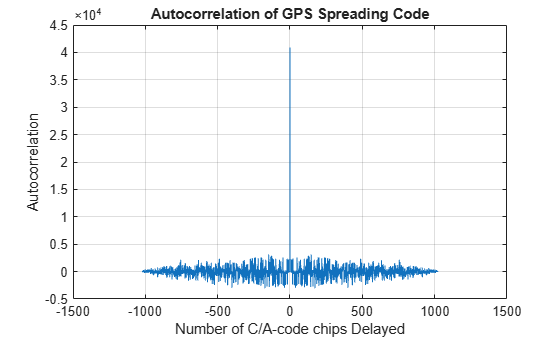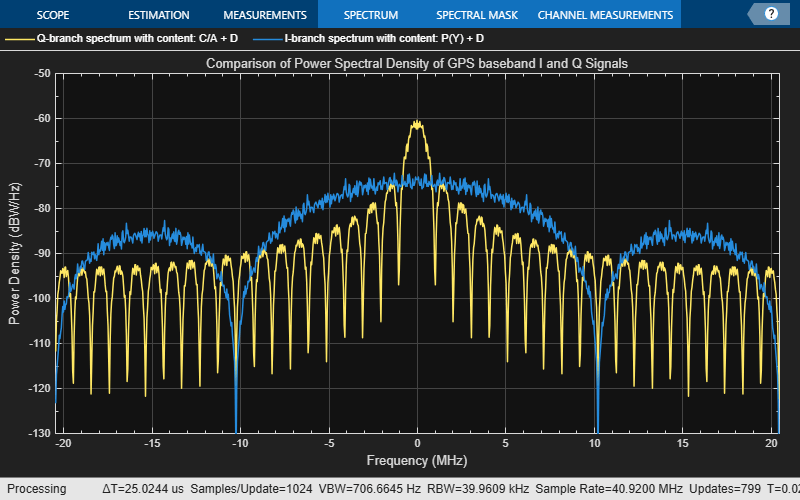### Further Exploration

This example uses a configuration object to generate GPS data bits and the navigation signal in the baseband. You can replace the property values in this configuration object and observe how the GPS data is generated. You can also change the ephemeris parameters with an existing real data set and pass those parameters into the CEI data set. Additionally, you can specify your own almanac file. If you are using your own almanac file, the week number in the almanac file and the week number in the configuration object must match.

Further, this example shows how to generate a GPS baseband waveform, which can be extended to generate an intermediate frequency (IF) waveform from the baseband waveform by multiplying a cosine signal on the in-phase branch and a sine signal on the quadrature-branch.

Additionally, this example shows how to generate a GPS waveform from one satellite, which can be combined along with multiple satellite PRN codes to get an integrated signal.

### Appendix

This example uses these data and helper files:

### Bibliography

 IS-GPS-200, Rev: L. NAVSTAR GPS Space Segment/Navigation User Segment Interfaces. May 14, 2020; Code Ident: 66RP1.